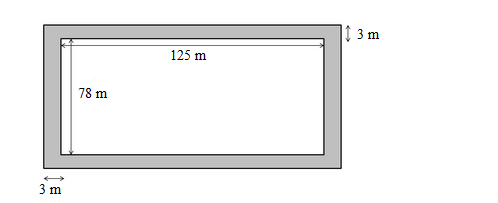# A rectangular plot measures 125 m by 78 m.`
Question:

A rectangular plot measures 125 m by 78 m. It has gravel path 3 m wide all around on the outside. Find the area of the path and the cost of gravelling it at Rs 75 per m2.

Solution:

The plot with the gravel path is shown in the figure.Area of the rectangular plot $=l \times b$

Area of the rectangular plot $=125 \times 78=9750 \mathrm{~m}^{2}$

Length of the park including the path = 125 + 6 = 131 m
Breadth of the park including the path = 78 + 6 = 84 m
Area of the plot including the path

$=131 \times 84$

$=11004 \mathrm{~m}^{2}$

Area of the path $=11004-9750$

= 1254 m2

Cost of gravelling 1 m2 of the path = Rs 75

$\therefore$ Cost of gravelling $1254 \mathrm{~m}^{2}$ of the path $=1254 \times 75$

$=$ Rs 94050